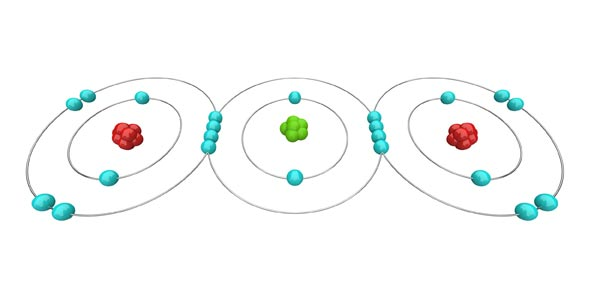# Ionic Substances And Naming

10 Questions | Attempts: 779SettingsWhat is an ionic substance? How is it named?

• 1.
What type of atoms (or ions) will form an ionic substance?
• A.

Two non-metals

• B.

Two metals

• C.

A metal ion and a non-metal ion

• 2.
What do the subscripts mean in a chemical formula? Example H2O
• A.

The subscript indicates the charge of the substance

• B.

The subscript indicates the number of atoms of each type that form the substance

• C.

The subscript indicates the location on the periodic table

• 3.
What type of ion do metals form?
• A.

Metals form positive cations. Example Na 1+

• B.

Metals form postiive anions. Example: Na 1+

• C.

Metals form negative anions. Example: Na1-

• 4.
Why are there 2 hydrogen and only 1 oxygen in water (H2O)?
• A.

Each hydrogen ion has a charge of 1+. This will balance the charge of 2- from the one oxygen ion

• B.

Each hydrogen has a charge of 2+ and the oxygen has a charge of 1-

• C.

The two hydrogen molecules have a combined charge of 2+ and the oxygen has a charge of 1-

• 5.
What type of bonds do salts form?
• A.

Salts are made up of two non-metals and form a covalent bond.

• B.

Salts are made up of a metal and non-metal and form an ionic bond.

• 6.
Compare the strength of an ionic bond to that of a covalent bond.
• A.

An ionic bond is very strong and therefore the substance will have a high melting and boiling point.

• B.

An ionic bond is weak and will have a low melting and boiling point

• 7.
Which of the following is the correct name for MgCl2?
• A.

Magnesium chloride

• B.

Magnesium (I) chloride

• C.

Magnesium (II) chloride

• 8.
What is the correct name for FeBr3?
• A.

Iron (I) bromide

• B.

Iron (III) bromide

• C.

Iron Bromide (III)

• D.

Iron Bromide

• 9.
What is correct formula for Calcium ion combined with a phosphate ion?
• A.

Ca3(PO4)2

• B.

CaPO4

• C.

CaP

• D.

Ca2(PO4)3

• 10.
What is the chemical formula for Potassium sulfate?
• A.

K(SO4)2

• B.

KSO4

• C.

K2SO4

## Related TopicsBack to top
×

Wait!
Here's an interesting quiz for you.